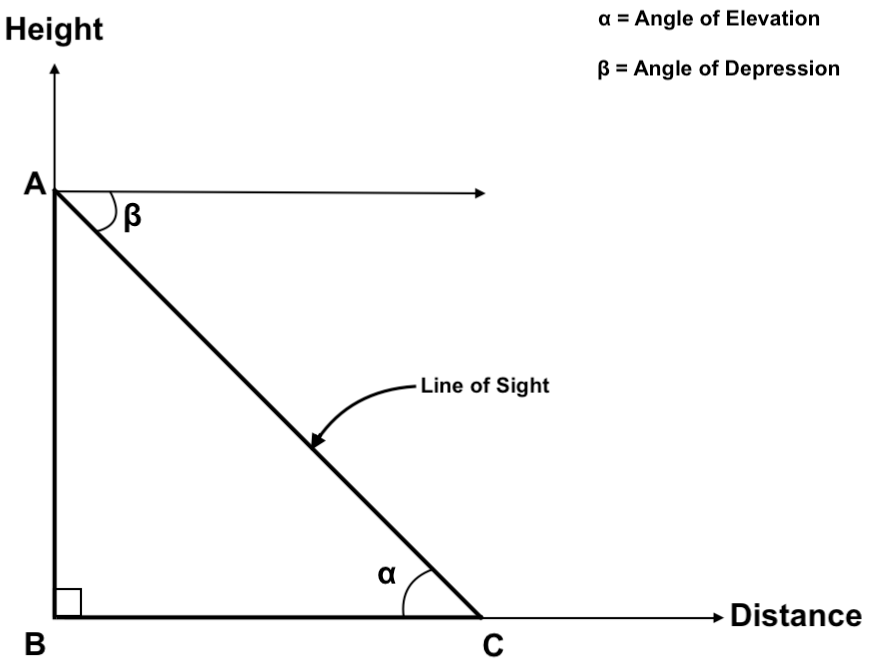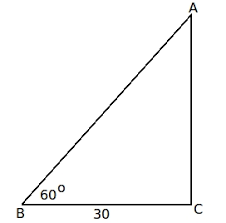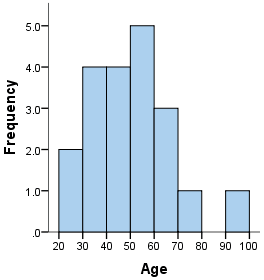SSC CGL 2020 Exam: Quantitative Abilities - Study24x7New to Study24X7 ?

# SSC CGL 2020 Exam: Quantitative Abilities

Updated on 15 February 2020SSC 2021 Preparation Strategie
Updated on 15 February 2020The quantitative abilities topic in SSC preparation is as important as general awareness. Both the topics can better prepare if the candidates preparing for competitive exam do practice both in English as well as Hindi.

Heights and Distances

• In trigonometry, we study the relationship between sides of the right-angle triangle and angle between the sides.Basics of Height and Distance:

1. Pythagoras theorem:

(Hypotenuse) 2 = (Base) 2+ (perpendicular or Height)2

2. Anle of Elevation

The angle of elevation is the angle between the base and the line of sight. In the above diagram, it is denoted by α.

3. Angle of Depression

If the object is at point C and viewer is watching it from point A and we draw an imaginary line from point A, then the angle between imaginary line and line of sight is called as angle of depression. In the above diagram, it is denoted by β.

Example: If a man is standing on the ground, near the ladder which is 30meter far from the wall and the ladder is standing along the wall at the angle of 600. Find the length of the ladder?

Solution: Here Angle of elevation, α = 600

Distance of person from wall=base=30 meterCos α = Base/ hypotenuse

Cos 600= 30/ length of the ladder

Length of the ladder= 60 meter

Histogram

Histogram is the pictorial representation of continuous data and is used to find out frequency distribution.

Construction of Histogram:

1. Collect the dataset.
2. Divide the data into equal intervals and assign it on the x-axis.
3. Allocate frequency of each interval in the dataset at the y-axis.
4. Connect them and draw the histogram.

Note: The Selection of interval of data is very important. It should not be very large or very small.

Calculation of frequency distribution:

The frequency distribution is calculated on the basis of the area of a rectangle covered under a particular interval.

Example:Here interval =10

X-axis shows different ages.

Y-axis shows the frequency of different age intervals.

Let us calculate the frequency distribution between 50- 60 years of age.

The value of this interval at the y-axis is 5 which gives the length of the rectangle and the base is 10.

= 5*10

=50

It means that there are 50 people in the range of age 50-60.

Frequency Polygon

• It is also a pictorial form to represent frequency distribution and to compare different data sets.
• It uses a line graph in place of bar graph, unlike histograms.

Method to draw frequency polygon:

1. Take the dataset.
2. Divide the data set into equal intervals.
3. Point out the intervals into the x-axis.
4. Find the frequency of each interval and mark it on the y-axis.
5. Point out the middle point of each interval corresponding to a particular frequency.
6. Middle point= (lower side of interval + higher side of interval)/2
7. Connect all the middle points marked according to frequency and form a line.
8.  After joining the line you will get a frequency polygon.NOTE: The value of frequency distribution is calculated by the total area covered under frequency polygon.

All the best to All the aspirants of SSC CGL 2020 !

Write a comment...Trending ArticlesBy CBSE CLASS 8By CBSE CLASS 10By CBSE CLASS 7By K-12-Commerce
Related Posts